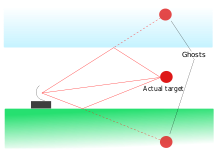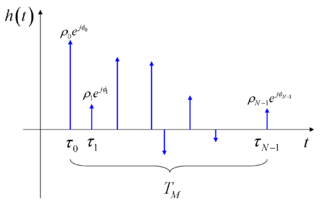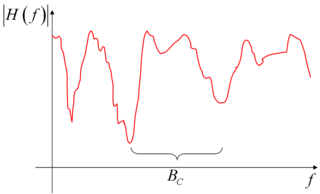# Multipath propagation

﻿
Multipath propagation

In wireless telecommunications, multipath is the propagation phenomenon that results in radio signals reaching the receiving antenna by two or more paths. Causes of multipath include atmospheric ducting, ionospheric reflection and refraction, and reflection from water bodies and terrestrial objects such as mountains and buildings.

The effects of multipath include constructive and destructive interference, and phase shifting of the signal. This causes Rayleigh fading. The standard statistical model of this gives a distribution known as the Rayleigh distribution.

Rayleigh fading with a strong line of sight content is said to have a Rician distribution, or to be Rician fading.

In facsimile and television transmission, multipath causes jitter and ghosting, seen as a faded duplicate image to the right of the main image. Ghosts occur when transmissions bounce off a mountain or other large object, while also arriving at the antenna by a shorter, direct route, with the receiver picking up two signals separated by a delay.Radar multipath echoes from an actual target cause ghosts to appear.

In radar processing, multipath causes ghost targets to appear, deceiving the radar receiver. These ghosts are particularly bothersome since they move and behave like the normal targets (which they echo), and so the receiver has difficulty in isolating the correct target echo. These problems can be overcome by incorporating a ground map of the radar's surroundings and eliminating all echoes which appear to originate below ground or above a certain height.

In digital radio communications (such as GSM) multipath can cause errors and affect the quality of communications. The errors are due to intersymbol interference (ISI). Equalisers are often used to correct the ISI. Alternatively, techniques such as orthogonal frequency division modulation and rake receivers may be used.

In a Global Positioning System receiver, Multipath Effect can cause a stationary receiver's output to indicate as if it were randomly jumping about or creeping. When the unit is moving the jumping or creeping is hidden, but it still degrades the displayed accuracy.

## Multipath propagation in wired media

Multipath propagation may also happen in wired media, especially in cases in which impedance mismatches cause signal reflections. A well-known example is power line communication.

High-speed power line communication systems usually employ multi-carrier modulations (such as OFDM or Wavelet OFDM) to avoid the intersymbol interference that multipath propagation would cause.

The ITU-T G.hn standard provides a way to create a high-speed (up to 1 Gigabit/s) local area network using existing home wiring (power lines, phone lines and coaxial cables). G.hn uses OFDM with a cyclic prefix to avoid ISI. Because multipath propagation behaves differently in each kind of wire, G.hn uses different OFDM parameters (OFDM symbol duration, Guard Interval duration) for each media.

## Mathematical modelingMathematical model of the multipath impulse response.

The mathematical model of the multipath can be presented using the method of the impulse response used for studying linear systems.

Suppose to transmit a single, ideal Dirac pulse of electromagnetic power at time 0, i.e.

x(t) = δ(t)

At the receiver, due to the presence of the multiple electromagnetic paths, more than one pulse will be received (we suppose here that the channel has infinite bandwidth, thus the pulse shape is not modified at all), and each one of them will arrive at different times. In fact, since the electromagnetic signals travel at the speed of light, and since every path has a geometrical length possibly different from that of the other ones, there are different air travelling times (consider that, in free space, the light takes 3 μs to cross a 1 km span). Thus, the received signal will be expressed by$y(t)=h(t)=\sum_{n=0}^{N-1}{\rho_n e^{j\phi_n} \delta(t-\tau_n)}$

where N is the number of received impulses (equivalent to the number of electromagnetic paths, and possibly very large), τn is the time delay of the generic nth impulse, and$\rho_n e^{j\phi_n}$ represent the complex amplitude (i.e., magnitude and phase) of the generic received pulse. As a consequence, y(t) also represents the impulse response function h(t) of the equivalent multipath model.

More in general, in presence of time variation of the geometrical reflection conditions, this impulse response is time varying, and as such we have

τn = τn(t)
ρn = ρn(t)
ϕn = ϕn(t)

Very often, just one parameter is used to denote the severity of multipath conditions: it is called the multipath time, TM, and it is defined as the time delay existing between the first and the last received impulses

TM = τN − 1 − τ0Mathematical model of the multipath channel transfer function.

In practical conditions and measurement, the multipath time is computed by considering as last impulse the first one which allows to receive a determined amount of the total transmitted power (scaled by the atmospheric and propagation losses), e.g. 99%.

Keeping our aim at linear, time invariant systems, we can also characterize the multipath phenomenon by the channel transfer function H(f), which is defined as the continuous time Fourier transform of the impulse response h(t)$H(f)=\mathfrak{F}(h(t))=\int_{-\infty}^{+\infty}{h(t)e^{-j 2\pi f t} d t}=\sum_{n=0}^{N-1}{\rho_n e^{j\phi_n} e^{-j2 \pi f \tau_n}}$

where the last right-hand term of the previous equation is easily obtained by remembering that the Fourier transform of a Dirac pulse is a complex exponential function, an eigenfunction of every linear system.

The obtained channel transfer characteristic has a typical appearance of a sequence of peaks and valleys (also called notches); it can be shown that, on average, the distance (in Hz) between two consecutive valleys (or two consecutive peaks), is roughly inversely proportional to the multipath time. The so-called coherence bandwidth is thus defined as$B_C \approx \frac{1}{T_M}$

For example, with a multipath time of 3 μs (corresponding to a 1 km of added on-air travel for the last received impulse), there is a coherence bandwidth of about 330 kHz.

Wikimedia Foundation. 2010.

### Look at other dictionaries:

• multipath propagation — šakotinis sklidimas statusas T sritis automatika atitikmenys: angl. multipath propagation vok. Mehrwegausbreitung, f rus. многолучевое распространение, n pranc. propagation par trajets multiples, f …   Automatikos terminų žodynas

• Multipath — may refer to: Multipath propagation in electromagnetic wave propagation Multipath I/O in operating system input/output subsystems Multipath routing in packet switching networks Multipath interference in wave transmission This disambiguation page… …   Wikipedia

• propagation par trajets multiples — šakotinis sklidimas statusas T sritis automatika atitikmenys: angl. multipath propagation vok. Mehrwegausbreitung, f rus. многолучевое распространение, n pranc. propagation par trajets multiples, f …   Automatikos terminų žodynas

• Multipath — En télécommunications sans fil, le multipath est un phénomène qui se produit lorsqu’un signal radio se propage par plusieurs chemins et est reçu sur une antenne. Les causes de l’atténuation multipath sont la réflexion sur l’ionosphère, réfraction …   Wikipédia en Français

• multipath — ˈməltə̇+ˌ adjective Etymology: multi + path, n. : of, relating to, or resulting from the propagation of electric waves over a number of different paths multipath transmission multipath phenomena * * * n …   Useful english dictionary

• multipath — 1. noun A wireless propagation phenomenon that results in a signal reaching the receiving antenna by two or more paths. 2. adjective Involving or pertaining to more than one path (route), as between nodes …   Wiktionary

• Non-line-of-sight propagation — Non line of sight (NLOS) or near line of sight is a term used to describe radio transmission across a path that is partially obstructed, usually by a physical object in the innermost Fresnel zone. Many types of radio transmissions depend, to… …   Wikipedia

• Radio propagation — is a term used to explain how radio waves behave when they are transmitted, or are propagated from one point on the Earth to another. [ H. P. Westman et al, (ed), Reference Data for Radio Engineers, Fifth Edition , 1968, Howard W. Sams and Co.,… …   Wikipedia

• Anomalous propagation — In Radio: Anomalous propagation includes different forms of electromagnetic wave propagation that are not encountered in a standard atmosphere. While technically the term includes propagation with larger losses than in standard atmosphere, in… …   Wikipedia

• Line-of-sight propagation — refers to electro magnetic radiation including light emissions traveling in a straight line. The rays or waves are diffracted, refracted, reflected, or absorbed by atmosphere and obstructions with material and generally cannot travel over the… …   Wikipedia